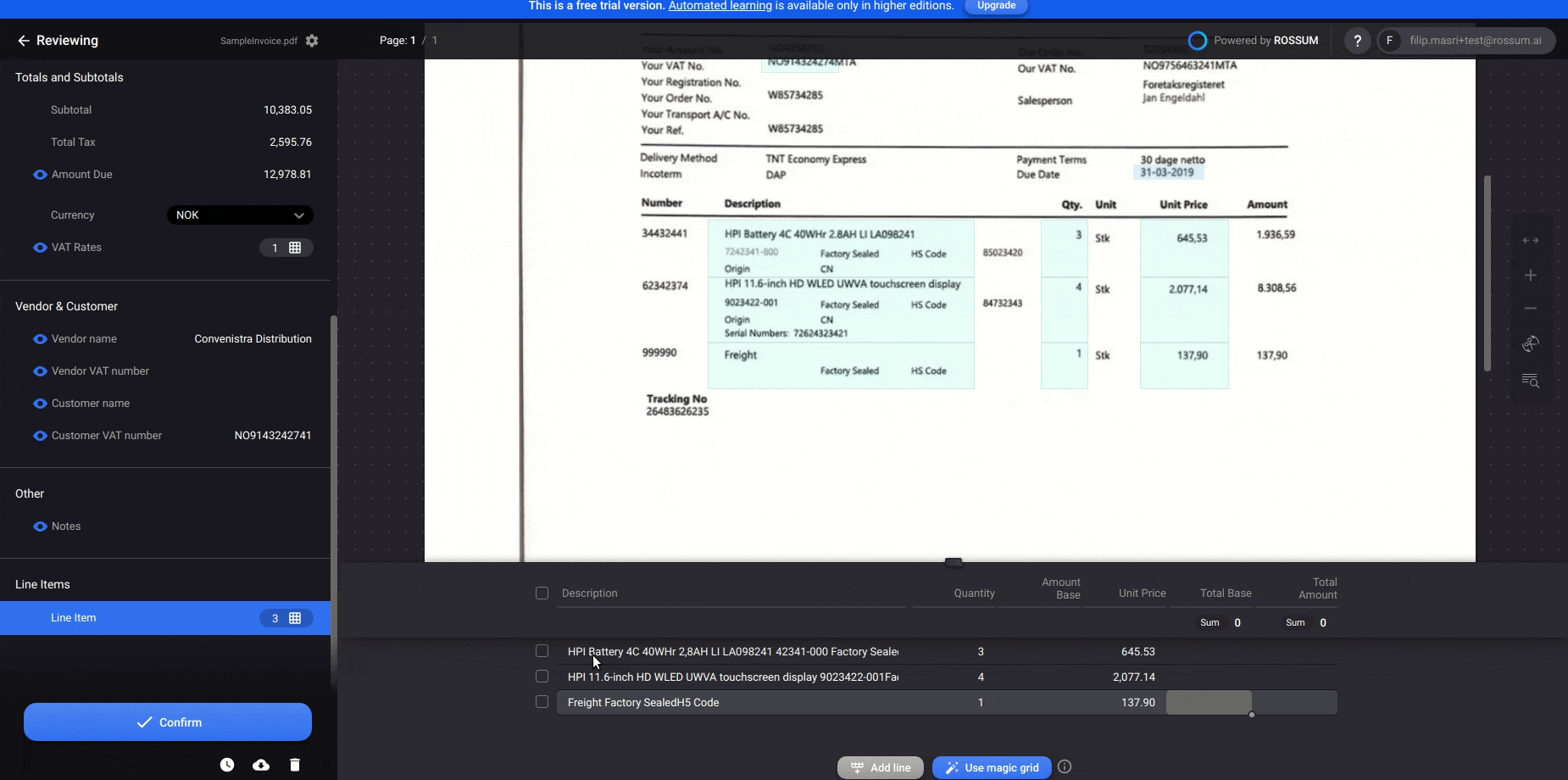# Switch total amount and total base columns based on totals on document

Sometimes it is difficult to decide whether a column in line items is a total amount with tax or without tax. This applies both to human and machine. The table column could have an ambiguous label (e.g. "Amount") and thus it would be difficult to classify. In order to decide correctly, you would compare the SUM of the line items column to the totals on the document. Based on the comparison you would decide whether to switch the some columns or not. And this is exactly what this function does.

In ideal case, Rossum would capture all the numbers in the columns correctly. Once having the values, you could already decide about switching the columns. This could be done even before an operator opens the document ("initialize" action in hook events). If some values were OCRed with errors, operator could correct them and based on the summations the columns could be switched after the user edited them ("user_update" action in hook events).

This code example assumes you have the following field IDs in your extraction schema:

• "amount_due" - for capturing the final value with tax to be paid
• "amount_total_base" - for capturing the final value without tax to be paid
• "item_amount_total" - for capturing the amount total with tax for line items
• "item_total_base" - for capturing the amount total without tax for line items

Moreover, the serverless function should be configured to get notifications on "user_update" and "initialize" action and the function should be assigned to some queue.

``````// This serverless function example can be used for annotation_content events
// (e.g. user_update action). annotation_content events provide annotation
// content tree as the input.
//
// The function below shows how to switch values of "Total amount" and
// "Total base" in the line items based on comparison to totals on the document.
//
// --- ROSSUM HOOK REQUEST HANDLER ---

// The rossum_hook_request_handler is an obligatory main function that accepts
// input and produces output of the rossum serverless function hook. Currently,
// the only available programming language is Javascript executed on Node.js 12 environment.
// @param {Object} annotation - see https://api.elis.rossum.ai/docs/#annotation-content-event-data-format
// @returns {Object} - the messages and operations that update the annotation content

exports.rossum_hook_request_handler = ({
annotation: {
content
}
}) => {
try {

const [amountDueDatapoint] = findBySchemaId(
content,
'amount_due',
);

const amountDue = parseFloat(amountDueDatapoint.content.normalized_value);

const [amountTotalBaseDatapoint] = findBySchemaId(
content,
'amount_total_base',
);

const amountTotalBase = parseFloat(amountTotalBaseDatapoint.content.normalized_value);

const amountTotalColumnDatapoints = findBySchemaId(
content,
'item_amount_total',
);

const amountTotalBaseColumnDatapoints = findBySchemaId(
content,
'item_total_base',
);

const messages = [];
const operations = [];

const lineItemsTotalBase = amountTotalBaseColumnDatapoints.reduce(function (sum, datapoint) { return sum + parseFloat(datapoint.content.normalized_value)}, 0);
const lineItemsTotal = amountTotalColumnDatapoints.reduce(function (sum, datapoint) { return sum + parseFloat(datapoint.content.normalized_value)}, 0);

if((amountTotalBase &&
amountTotalBase != lineItemsTotalBase &&
amountTotalBase == lineItemsTotal) ||
(amountDue &&
amountDue != lineItemsTotal &&
amountDue == lineItemsTotalBase)){

for(var i = 0; i < amountTotalBaseColumnDatapoints.length; i++){

operations.push({op: 'replace', id: amountTotalBaseColumnDatapoints[i].id, schema_id: amountTotalColumnDatapoints[i].schema_id, value: {content: amountTotalColumnDatapoints[i].content}})
operations.push({op: 'replace', id: amountTotalColumnDatapoints[i].id, schema_id: amountTotalBaseColumnDatapoints[i].schema_id, value: {content: amountTotalBaseColumnDatapoints[i].content}})
}

}

// Return messages and operations to be used to update current annotation data
return {
messages,
operations,
};
} catch (e) {
// In case of exception, create and return error message. This may be useful for debugging.
const messages = [
createMessage('error', 'Serverless Function: ' + e.message)
];
return {
messages,
};
}
};

// --- HELPER FUNCTIONS ---

// Return datapoints matching a schema id.
// @param {Object} content - the annotation content tree (see https://api.elis.rossum.ai/docs/#annotation-data)
// @param {string} schemaId - the field's ID as defined in the extraction schema(see https://api.elis.rossum.ai/docs/#document-schema)
// @returns {Array} - the list of datapoints matching the schema ID

const findBySchemaId = (content, schemaId) =>
content.reduce(
(results, dp) =>
dp.schema_id === schemaId ?
[...results, dp] :
dp.children ?
[...results, ...findBySchemaId(dp.children, schemaId)] :
results,
[],
);

// Create a message which will be shown to the user
// @param {number} datapointId - the id of the datapoint where the message will appear (null for "global" messages).
// @param {String} messageType - the type of the message, any of {info|warning|error}. Errors prevent confirmation in the UI.
// @param {String} messageContent - the message shown to the user
// @returns {Object} - the JSON message definition (see https://api.elis.rossum.ai/docs/#annotation-content-event-response-format)

const createMessage = (type, content, datapointId = null) => ({
content: content,
type: type,
id: datapointId,
});

// Replace the value of the datapoint with a new value.
// @param {Object} datapoint - the content of the datapoint
// @param {string} - the new value of the datapoint
// @return {Object} - the JSON replace operation definition (see https://api.elis.rossum.ai/docs/#annotation-content-event-response-format)

const createReplaceOperation = (datapoint, newValue) => ({
op: 'replace',
id: datapoint.id,
value: {
content: {
value: newValue,
},
},
});
``````